3 篇文章 1 订阅

# 1.0 引言

## 1.1 需要补充的概率论知识

• 随机变量(random variable)的概念
•  期望(expectation)的概念与定义
• 伯努利分布(bernouli distribution)
• 二项式分布(binomial distribution)
• 泰勒展开(Talyor expansion)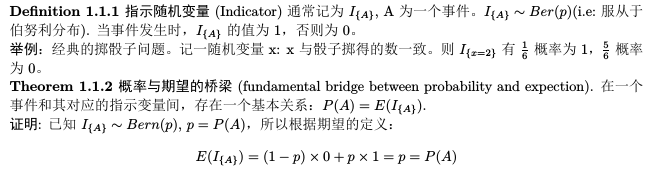## 2 霍夫丁不等式理解

### 2.1霍夫丁不等式与机器学习的关系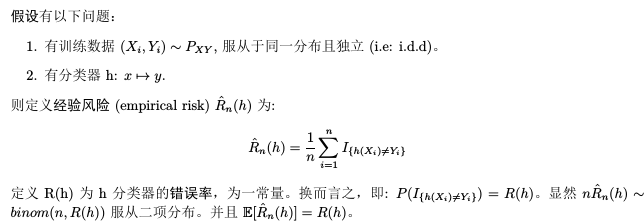### 2.2 霍夫丁不等式数学公式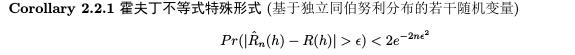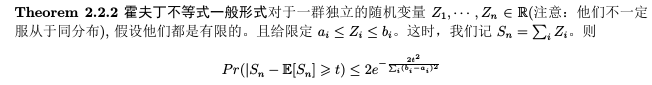## 3 霍夫丁不等式的推导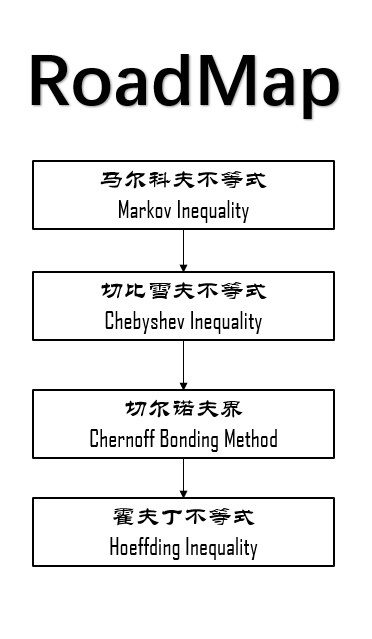### 3.1 马尔科夫不等式的推导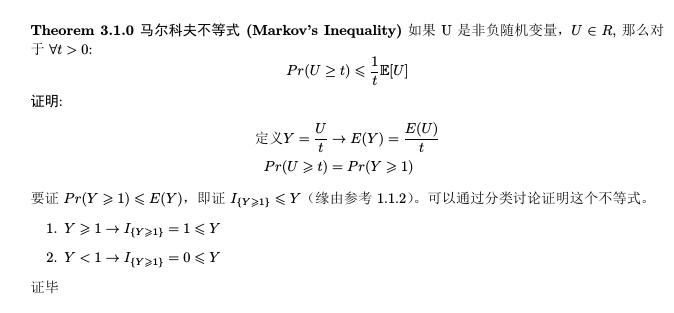### 3.2 切比雪夫不等式的推导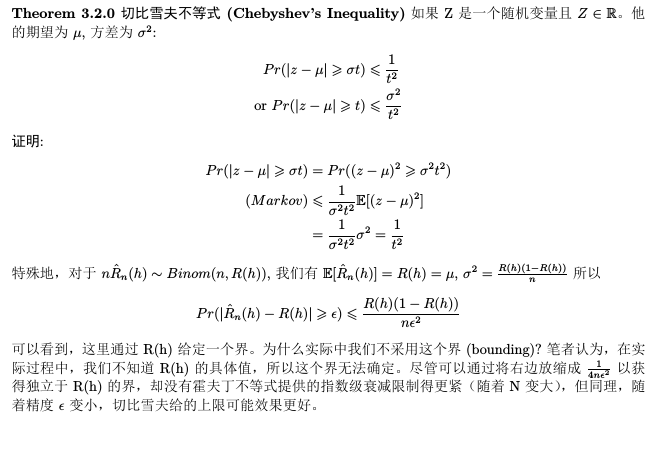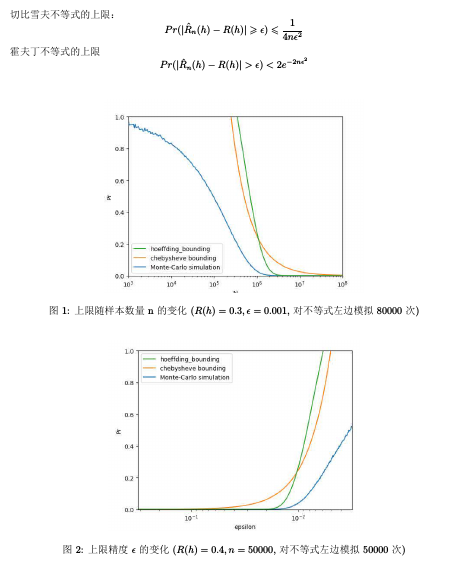### 3.3 切尔诺夫界的推导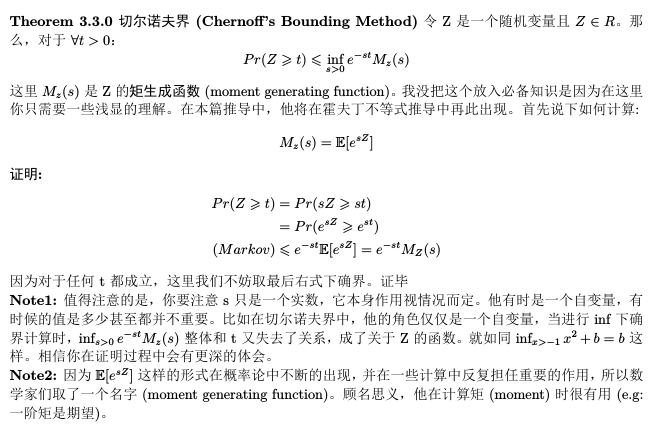### 3.4 霍夫丁不等式的推导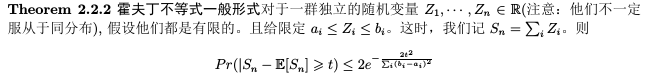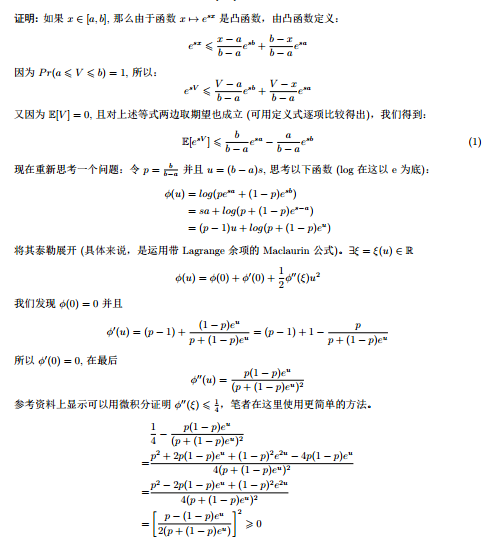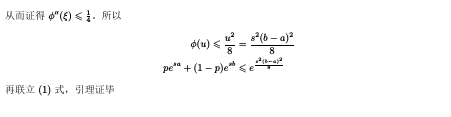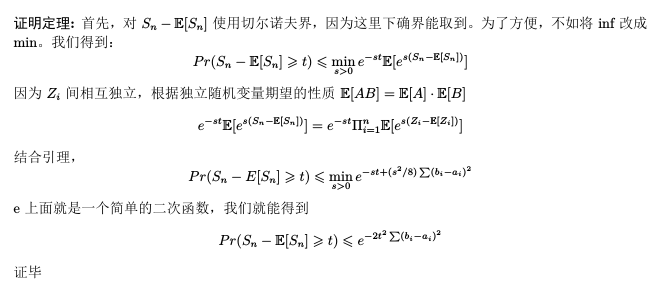### 5 附录

5.A 模拟程序(python)

from scipy import stats
import numpy as np
import matplotlib.pyplot as plt

if __name__ == "__main__":
N = np.array(np.power(10, np.arange(3, 8, 0.02)), dtype=np.int32)
p = 0.3
epsilon = 0.001
Pr = np.zeros(N.shape)
size = 80000
i = 0
for n in N:
print(n)
X = stats.binom.rvs(n, p, size=size)
Pr[i] = np.count_nonzero(np.abs(X / n - p) > epsilon) / size
i += 1

chebysheve_bounding = 1 / (4 * N * epsilon * epsilon)
hoeffding_bounding = 2 * np.exp(-2 * N * epsilon * epsilon)
fig = plt.figure()

ax.plot(N, Pr, label="Monte-Carlo simulation")
ax.plot(N, chebysheve_bounding, label="chebysheve bounding")
ax.plot(N, hoeffding_bounding, label="hoeffding_bounding")
handles, labels = ax.get_legend_handles_labels()
ax.legend(handles[::-1], labels[::-1])
plt.xscale('log')
plt.xlim(10 ** 3, 10 ** 8)
plt.ylim(0, 1)

plt.xlabel("N")
plt.ylabel("Pr")

plt.show()

from scipy import stats
import numpy as np
import matplotlib.pyplot as plt

if __name__ == "__main__":
n = 10000
p = 0.4
epsilon = np.power(10, np.arange(-3, -1, 0.001))
Pr = np.zeros(epsilon.shape)
size = 5000
i = 0
for e in epsilon:
print(e)
X = stats.binom.rvs(n, p, size=size)
Pr[i] = np.count_nonzero(np.abs(X / n - p) > e) / size
i += 1

chebysheve_bounding = 1 / (4 * n * epsilon * epsilon)
hoeffding_bounding = 2 * np.exp(-2 * n * epsilon * epsilon)
fig = plt.figure()

ax.plot(epsilon, Pr, label="Monte-Carlo simulation")
ax.plot(epsilon, chebysheve_bounding, label="chebysheve bounding")
ax.plot(epsilon, hoeffding_bounding, label="hoeffding_bounding")
handles, labels = ax.get_legend_handles_labels()
ax.legend(handles[::-1], labels[::-1])
plt.xscale('log')
plt.xlim(10 ** -1, 10 ** -3)
plt.ylim(0, 1)

plt.xlabel("epsilon")
plt.ylabel("Pr")

plt.show()


02-03968905-245556
02-182380
09-147941
12-01239
08-262367
10-221万+
03-085092
08-212万+
11-08733
06-223万+
02-108396
06-13
09-04543
12-01358
07-082486
07-317284点击重新获取扫码支付余额充值